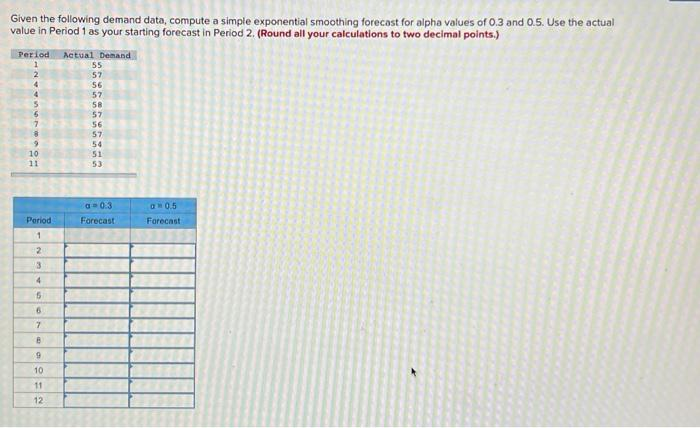Home / Expert Answers / Operations Management / given-the-following-demand-data-compute-a-simple-exponential-smoothing-forecast-for-alpha-values-o-pa454

# (Solved): Given the following demand data, compute a simple exponential smoothing forecast for alpha values o ...Given the following demand data, compute a simple exponential smoothing forecast for alpha values of \( 0.3 \) and \( 0.5 \). Use the actual value in Period 1 as your starting forecast in Period 2, (Round all your calculations to two decimal points.)

We have an Answer from Expert